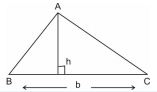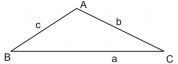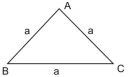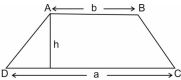# Herons Formula class 9 Notes Mathematics## myCBSEguide App

CBSE, NCERT, JEE Main, NEET-UG, NDA, Exam Papers, Question Bank, NCERT Solutions, Exemplars, Revision Notes, Free Videos, MCQ Tests & more.

CBSE class 9 Mathematics Chapter 12 Herons Formula notes in PDF are available for free download in myCBSEguide mobile app. The best app for CBSE students now provides Herons Formula class 9 Notes latest chapter wise notes for quick preparation of CBSE board exams and school based annual examinations. Class 9 Mathematics notes on Chapter 12 Herons Formula are also available for download in CBSE Guide website.

## CBSE Guide Herons Formula class 9 Notes

CBSE guide notes are the comprehensive notes which covers the latest syllabus of CBSE and NCERT. It includes all the topics given in NCERT class 9 Mathematics text book. Users can download CBSE guide quick revision notes from myCBSEguide mobile app and my CBSE guide website.

## 10 Mathematics notes Chapter 12 Herons Formula

Download CBSE class 9th revision notes for Chapter 12 Herons Formula in PDF format for free. Download revision notes for Herons Formula class 9 Notes and score high in exams. These are the Herons Formula class 9 Notes prepared by team of expert teachers. The revision notes help you revise the whole chapter in minutes. Revising notes in exam days is on of the best tips recommended by teachers during exam days.

CBSE Class 09 Mathematics
Revison Notes
CHAPTER 12
HERON’S FORMULA

1. Area of a Triangle – by Heron’s Formula

2. Application of Heron’s Formula in finding Areas of Quadrilaterals

• Triangle with base ‘b’ and altitude ‘h’ is• Area of an isosceles triangle whose equal side is  =  square units
• Triangle with sides a, b and c

(i) Semi perimeter of triangle s =

(ii) Area=sq. unit• Equilateral triangle with side ‘a’

Perimeter =  units

Altitude =  units• Rectangle with length , breadth

Perimeter =

Area =

• Square with side

Perimeter =  units

Area =   sq. units

Area =  sq. units

• Parallelogra with length , breadth  and height

Perimeter =

Area =

• Trapezium with parallel sides ‘a’ & ‘b’ and the distance between two parallel sides as ‘h’.## Herons Formula class 9 Notes

• CBSE Revision notes for Class 9 Mathematics PDF
• CBSE Revision notes Class 9 Mathematics – CBSE
• CBSE Revisions notes and Key Points Class 9 Mathematics
• Summary of the NCERT books all chapters in Mathematics class 9
• Short notes for CBSE class 9th Mathematics
• Key notes and chapter summary of Mathematics class 9
• Quick revision notes for CBSE board exams

## CBSE Class-10 Revision Notes and Key Points

Herons Formula class 9 Notes. CBSE quick revision note for Class-10 Mathematics, Chemistry, Maths, Biology and other subject are very helpful to revise the whole syllabus during exam days. The revision notes covers all important formulas and concepts given in the chapter. Even if you wish to have an overview of a chapter, quick revision notes are here to do if for you. These notes will certainly save your time during stressful exam days.

To download Herons Formula class 9 Notes, sample paper for class 9 Mathematics, Social Science, Science, English Communicative; do check myCBSEguide app or website. myCBSEguide provides sample papers with solution, test papers for chapter-wise practice, NCERT solutions, NCERT Exemplar solutions, quick revision notes for ready reference, CBSE guess papers and CBSE important question papers. Sample Paper all are made available through the best app for CBSE students and myCBSEguide website.